# Degree Centrality of a Graph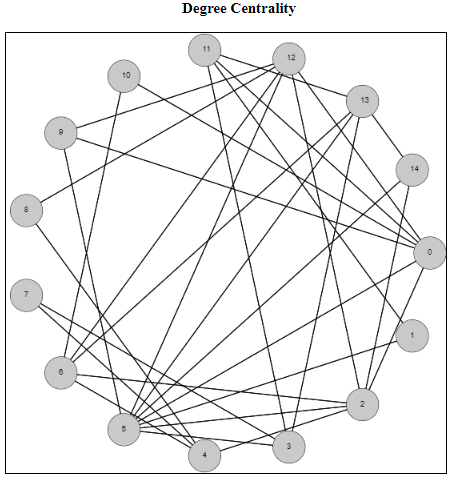I wanted to spend some time on centrality measures of a graph. These are measurements of how important each node (or edge) is to the overall graph. But how do we define, or determine, importance? There is no unique way to answer this question, so there are varying metrics for measuring centrality. Which one you choose depends on several factors including how many other nodes of the graph are included, as well as the run time of the metrics you’re considering.

I have just published a script focusing on the degree centrality metric. The degree centrality metric is called a “walk metric” because it determines how important a node is by how many other nodes that can be reached by walks of up to a certain length. Lets look at the definition of the degree of a node to see if we can understand why it is called a walk metric.

In an undirected graph G = (V, E), the degree of a node u [in] V is the |{v | (u, v) [in] E}|. This is the size of the set of nodes that are connected to node u via a single edge. Another way of describing a single edge is a walk of length one. So the degree metric measures the importance of a node by the number of unique walks of length one.

The normalized degree centrality of a node v in a graph G = (V,E) measures how many nodes are connected to the node v, compared to the maximum possible number of edges that can be connected to this node. Because we are dealing with simple undirected graphs (at most a single edge between any two distinct vertices), this maximum possible number will always be |V – 1|. So the normalized degree can be calculated by dividing the degree of the node (the number of nodes it is connected to) by |V – 1|.

So for the example above, the node 0 has degree 6 because it is connected to nodes 2, 5, 9, 10, 11, and 12. There are 15 total nodes in this graph, so to calculate the normalized degree centrality of the node 0, it will be 6 / 14, which rounds to 0.428571.

To see more examples and to help answer questions, check out the script in my examples section on degree centrality

# Tarjan’s Strongly Connected Components Algorithm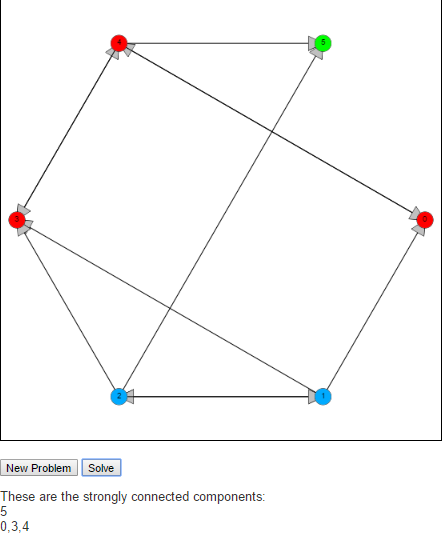A strongly connected component of a graph is a subgraph S of G where every pair of nodes, u and v in S there is a path from u to v and a path from v to u.

To find these strongly connected components we implement Tarjan’s algorithm. The idea behind Tarjan’s algorithm is to begin by running a depth first search from an arbitrary node in the graph, labeling nodes reachable from this start node in the order they are reached. The algorithm is also interested in the “oldest” node that could be reached by a given node. This is indicated by the keeping track of the lowest label that can be reached from that node. We will call the first property label(v) and the second lowlink(v).

When the algorithm starts label(v) is the same as lowlink(v) whenever a node is discovered. As the algorithm is executed, the DFS is being run on each discovered node, which in turn updates the lowlink(v) property telling of (older) nodes that can be reached. If an older node can be reached, then we update lowlink. If we reach a node that cannot connect to any older nodes after the DFS call, i.e if label(v) is the same a lowlink(v), then this means that this node does not have a path to any node with a lower label. So this node will be the first node of a new strongly connected component.

Feel free to check it out an let me know what you think in the comments below.

# Longest Common Subsequence

Suppose you and I each had an ordered list of items and we were interested in comparing how similar those lists are. One calculation we can perform on these two strings is the Longest Common Subsequence. A sequence X is an ordered list of elements <x1, …, xn>. A subsequence Z is another sequence where (1) Each element of Z is also an element of X and (2) The elements of Z occur in the same order (in Z) as they do in X.

Note that we do not say that the elements of Z need to be a continuous block of elements. If this were true we would be defining a substring. So as an example, suppose we have as an initial string,
X = C, O, M, P, U, T, E, R.
Then the following are all subsequences:
Z1 = C, M, U, T, R
Z2 = C, O, M, P
Z3 = U, T, E, R
Z4 = O, P, T, E

I will note that Z2 and Z3 are also substrings since they contain continuous sets of characters.

The length of a substring is simply the number of characters it contains. So X has length 8, Z1 has length 5, Z2, Z3 and Z4 have length 4.

Suppose now that we had a second string, Y = P, R, O, G, R, A, M, M, E, R and are interested in the longest common subsequence between the two. We can do that by observing that there is a bit of recursion going on with this question. What I mean by that is that asking the question of “What is the longest common subsequence between X and Y” is the same as asking “What is the longest common subsequence between X and Y once we have seen 8 characters of X and 10 characters of Y”

There are three possible ways to answer this question.

If X<sub>8</sub> is not equal to Y<sub>10</sub>, then the answer to this will be the same as the maximum of the pair X<sub>7</sub>, Y<sub>10</sub> and the pair X<sub>8</sub>, Y<sub>9</sub>.
If we reach a situation where we reach the beginning of either string, we are forced to answer 0 to that question.

Then the function has the following look:

LCS(Xi, Yj) =
 0, if i is 0 or j is 0 1 + LCS(Xi-1, Yj-1) if Xi equals Yj max(LCS(Xi-1, Yj), LCS(Xi, Yj-1))

Below is a table showing how we would solve the problem mentioned.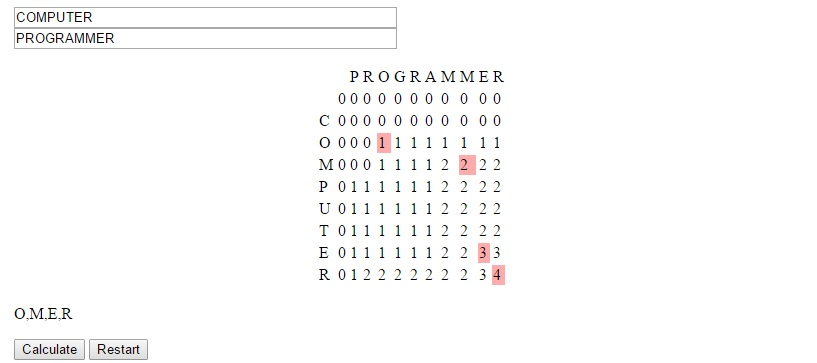The strategy used to devise this concept is called dynamic programming. It is useful we can solve larger problems by solving overlapping subproblems, as was the case here. In this situation we generally can store the data in a table form and avoid re-solving subproblems for which many larger problems will be dependent.

You can see this algorithm in action at LEARNINGlover.com: Longest Common Subsequece. Let me know what you think.

# Independent Set Puzzles

In this post, I want to return to the idea of NP-Complete problems. There is a more technical, more formal definition that I can refer you to, but I like to refer to the images from Garey and Johnson’s “Computers and Intractability: A Guide to the Theory of NP Completeness”. The images helps to understand the difficulty of NP-Complete problems by presenting two images. The first image shows a single individual speaking to someone saying that he has been unable to solve the problem. The second image shows that same individual speaking to the same person behind the desk, but saying that not only was he unable to solve the problem but neither was a long line of people. The theory of NP Complete problems revolves around the concept that if an efficient algorithm exists for an NP-Complete problem, then an efficient algorithm exists for all problems in the class NP.

Today, I would like to present a puzzle I created to play with the Independent Set problem. This is the problem where we are given a graph G = (V, E) and are asked to find a maximum set of vertices S such that there is no edge in the graph G between any two vertices in S. The decision version (the problem asking whether there is an independent set of size k) of this problem is NP-Complete, so the known algorithms for problem either have a slow running time, or do not solve it exactly.

This problem is very related to another puzzle I posted last year called the clique problem. In fact, Karp originally proved that Clique was NP-Complete by showing that if Clique could be solved efficiently, then Independent Set could be solved efficiently. He did this by constructing a second graph [G bar], called the compliment of G (containing the same vertices in G, along with the edges that are not present in G. Any edge present in G will not be present in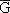). Then he showed that the nodes representing a maximum clique in G would represent a maximum independent set in. He had already shown that Independent Set was NP-Complete, which meant that both Independent Set and Clique were among the most difficult problems within the class known as NP.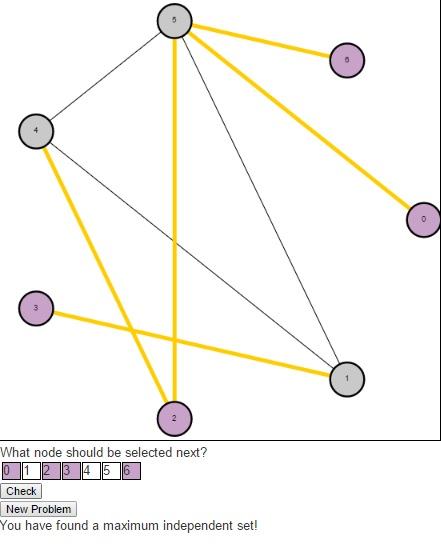The puzzle begins with an undirected graph and asks users to find a maximum independent set. Users should click on the numbers in the table below the graph indicating the nodes they wish to select in their independent set (purple indicates that the node is selected, gray indicates that it is not). Once a user have a potential solution, they can press the “Check” button to see if their solution is optimal. If a user is having trouble and simply wishes to see the maximum independent set, they can press the “Solve” button. And to generate a new problem, users can press the “New Problem” button.As a result of this relationship between the Clique problem and the Independent Set problem, the Bron-Kerbosch algorithm that was used to find maximum cliques previously can also be used here.

# Lets Learn About XOR Encryption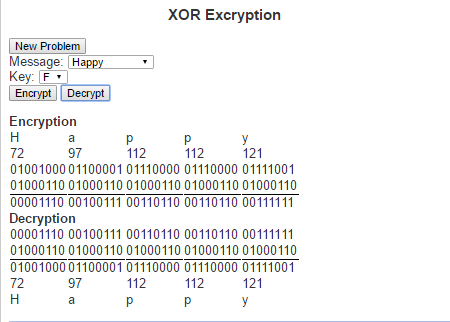One of the more common things about this generation is the constant desire to write up (type) their thoughts. So many of the conversations from my high school days were long lasting, but quickly forgotten. Today’s generation is much more likely to blog, tweet, write status updates or simply open up a notepad file and write up their thoughts after such a conversation.

When we feel that our thoughts are not ready for public eyes (maybe you want to run your idea by the Patent and Trademark Office before speaking about it) we may seek some form of security to ensure that they stay private. An old fashioned way of doing this was to write in a diary and enclosed it within a lock and key. The mathematical field of encryption also tries to grant privacy by encoding messages so that only people with the necessary information can read them.

The type of encryption I want to speak about today is called XOR encryption. It is based on the logical operation called “exclusive or” (hence the name XOR). The exclusive or operation is true between two logical statements if exactly one of the two statements is true, but not both statement. This can be represented with the following truth table

 Input 1 Input2 XOR Result T T F T F T F T T F F F

XOR Encryption is particularly useful in this day and age because we every character we type is understood by the computer as a sequence of zeros and ones. The current standard encoding that is used is Unicode (also known as UTF-8). Under this encoding the letter ‘a’ is represented as the binary string ‘01100001’. Similarly every letter, number and special character can be represented as its own binary string. These binary strings are just an assignment of numbers to these characters so that we can to help represent them in the computer. The numbers can the be thought of in base 10, which is how we generally think about numbers, or in base 2 which is how computers generally work with numbers (or a number of other ways). The way we would use these binary strings in encoding is first by translating a text from human-readable text to machine readable text via its binary string. For example, the word “Invincible”, we would get the following binary strings:

 Letter Unicode in base 10 Unicode in base 2 I 73 01001001 n 110 01101110 v 118 01110110 i 105 01101001 n 110 01101110 c 99 01100011 i 105 01101001 b 98 01100010 l 108 01101100 e 101 01100101

To encrypt the message we need a key to encode the message and will simply perform an XOR operation on the key and every character in the string. Similarly, do decrypt the message we perform XOR operation on the key and every character in the encoded message. This means that the key (much like a normal key to a diary) must be kept private and only those whom the message is to be shared between have access to it.

Here is a link to the script where you can check out XOR Encrpytio. Try it out and let me know what you think.